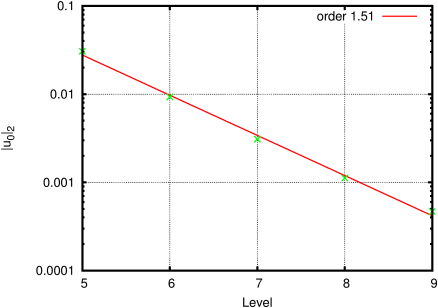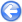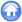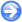### 12.1PASS: Oscillations in a parabolic container

Author
Stéphane Popinet
Command
sh parabola.sh parabola.gfs
Version
1.3.1
Required files
parabola.sh error.ref
Running time
25 seconds

Analytical solutions for the damped oscillations of a liquid in a parabolic container have been derived by Sampson et al [40, 25]. Figure 139 illustrates the numerical and analytical solutions at t = 1500 seconds. Wetting and drying occur at the two moving contact points and hydrostatic balance is approached as time passes.

Figure 140 gives the analytical and numerical solutions for the horizontal component of velocity (which is spatially constant). Figures 141 and 142 give the relative errors in surface elevation and horizontal velocity respectively, as functions of spatial resolution.

The errors are small and larger-than-first-order convergence rates are obtained.

 Figure 139: Solution at t = 1500 seconds. Six levels of refinement.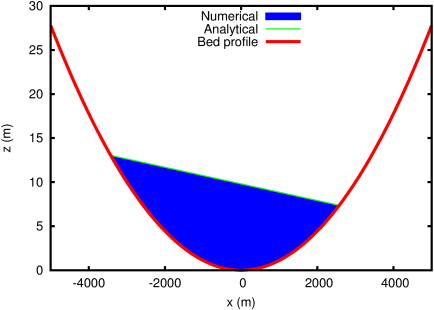Figure 140: Time evolution of the (spatially constant) horizontal velocity. Seven levels of refinement.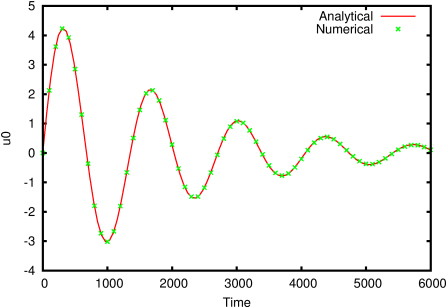Figure 141: Evolution of the relative elevation error norms as functions of resolution.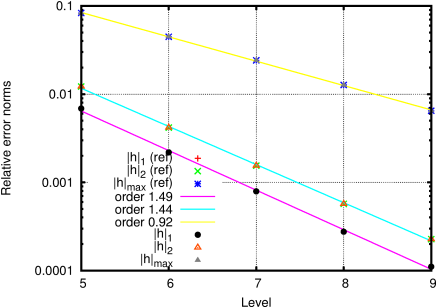Figure 142: Evolution of the relative velocity error L2-norm as a function of resolution.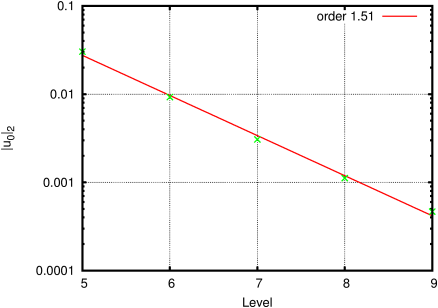#### 12.1.1PASS: Parabolic container with embedded solid

Author
Hyunuk An, Soonyoung Yu and Stéphane Popinet
Command
sh ../parabola.sh solid.gfs
Version
120314
Required files
error.ref
Running time
4 minutes 6 seconds

Same test case but with a slightly-inclined, narrow channel defined using embedded solid boundaries.

 Figure 143: Time evolution of the (spatially constant) horizontal velocity. Seven levels of refinement.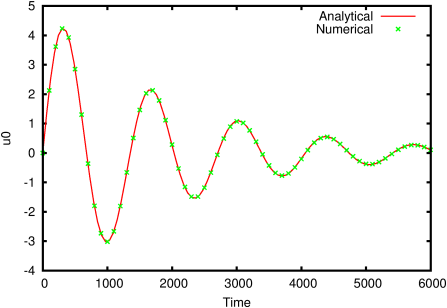Figure 144: Evolution of the relative elevation error norms as functions of resolution.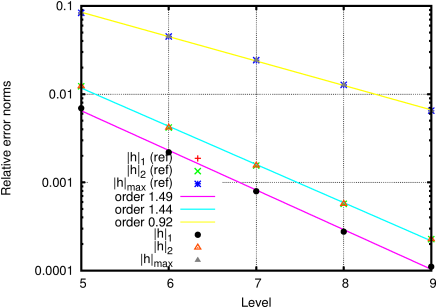Figure 145: Evolution of the relative velocity error L2-norm as a function of resolution.